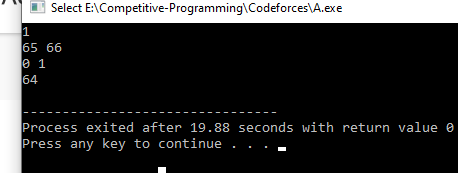# (HELP) TCS DIGITAL CODING QUESTION - AUCTION NUMBER

Actually for this test case:
1
A B
0 1

but according to your code it is 64#include <bits/stdc++.h>
#define int long long
using namespace std;

int32_t main() {

``````int dist;
cin >> dist;

char st,end;
cin >> st >> end;

int allowed= (end-st)+1;

int first,second;
cin >> first >> second;

int decimal=second-first+1;

int ans=dist*pow(allowed,2);

int ans2=pow(decimal,4)-(decimal-1)*decimal*4-decimal;
ans*=ans2;

cout << ans << endl;

return 0;
``````

}

1 Like

Thank you very much, Can i know the logic for ans2 variable plz

@shinigaami is this oncampus event ??? I am very confused about what is going on TCS hiring through codevita. I am selected for ninja profile through codevita, but want upgrade to digital.

See the ans2 variable is for the 4th part of the number plate
So for calculating the total possible permutations first we calculate the total permutations which will be (decimal)^4 after that we have to subtract the special numbers.
So lets say we have 0 1 as input then we want to generate a four digit number with 0’s and 1’s then special number in this case would be 0001, 0010,0100,1000 ,0000 and same goes with 1.
So total permutation were we have 3 zeros or 3 ones is 4*(decimal-1)decimal and then we subtract the numbers which have all zeros and all ones hence the formula becomes
ans2=(decimal)^4-4
(decimal-1)*decimal-decimal

P.S: I had this same question in TCS NQT exam and submitted the same but it was still not accepted as one of the private test cases wasn’t passed. So if you find anything wrong with this code please do mention. Somehow I still got shortlisted for the interview process and it is scheduled on 13th October and I still dont know what is wrong with my code hence if you find any edge cases that are not handled in this code please do mention.

1 Like

That is great explanation thanks, and No, I am not able to find any edge cases where it might be wrong, i am shocked that in tcs nqt this type of sum was there, where full output is necessary.

Anyways thanks! for the code and explanation.

1 Like

Sorry to disturb you again, but my brother is asking why did you do (decimal - 1) I explained him decimal*4 but was not able to explain decimal - 1lets say we have 3 numbers 0,1 and 2. Now count the total possible special permutations for 0
0001,0010,0100,1000 //4 cases
0002// 4 cases
same goes if we count for 1 and 2.

Lets take another example where we have 4 numbers 0,1,2,3 //hence decimal=4
count possible special permutations for 0
0001//4 cases
0002//4 cases
0003//4 cases
total cases for 0=(decimal-1)4
same goes for 1,2,3
hence total cases = decimal
[(decimal-1)*4]
and at last we subtract those cases which have all the same numbers which are 0000,1111,2222,3333 in this case

1 Like

NOICE!!!1 Like